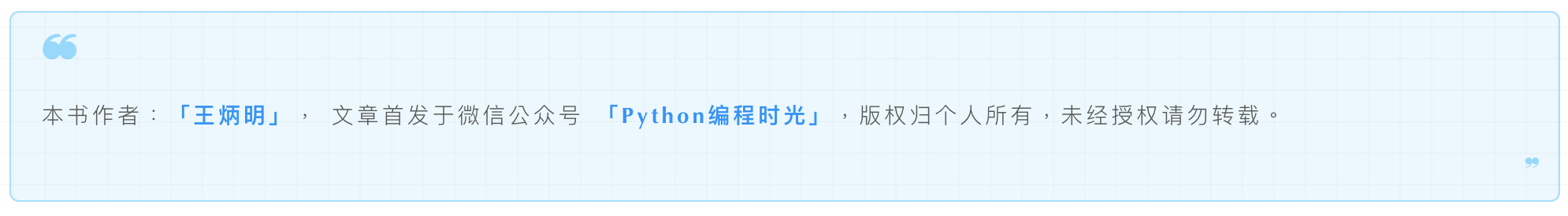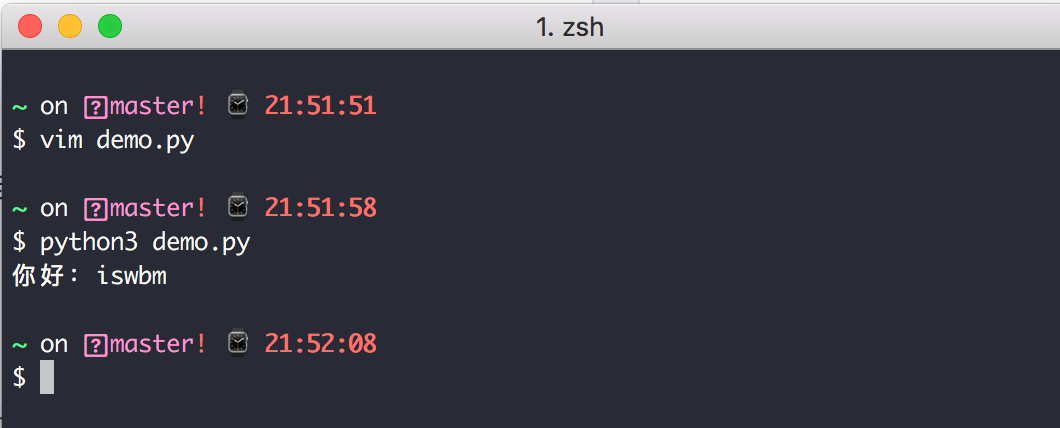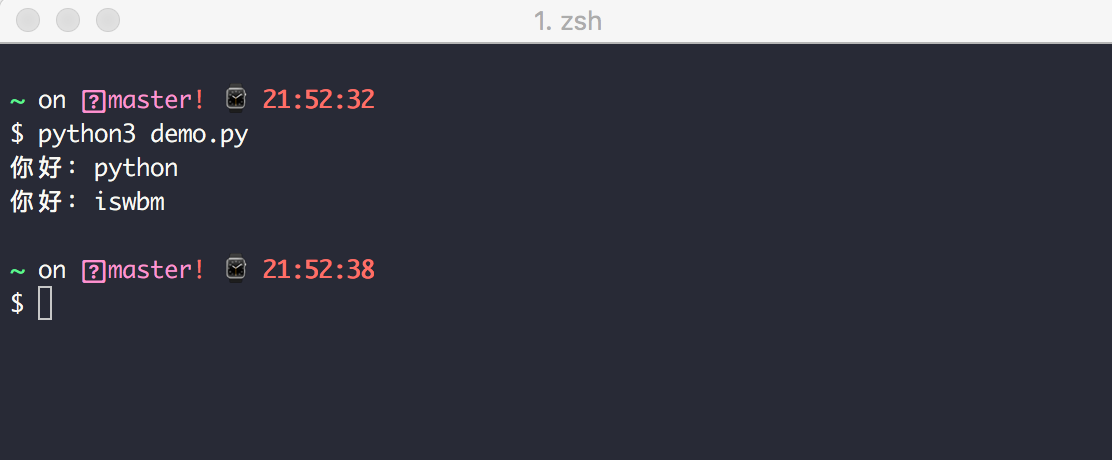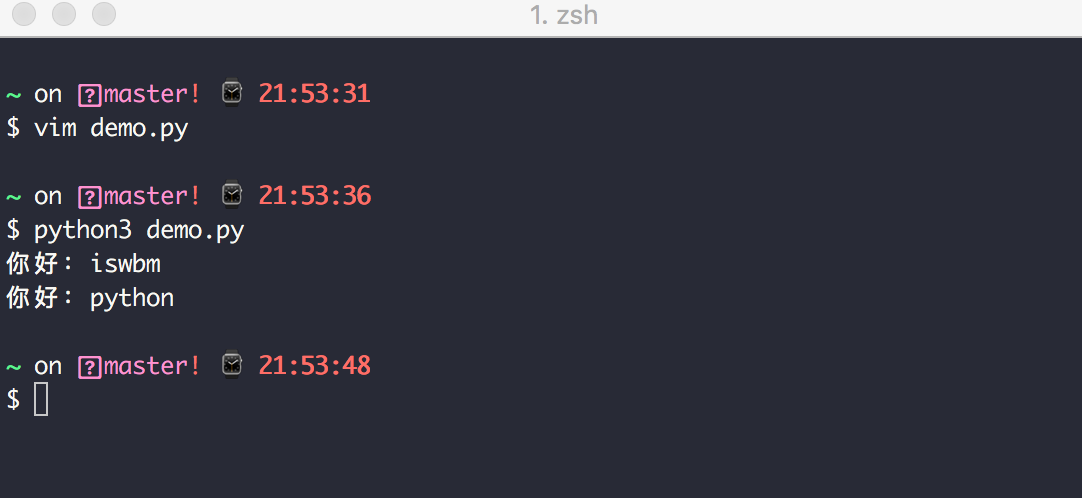# 7.12 非常好用的调度模块¶Python 自带一个调度器模块`sched`，它能为你实现优先级队列/延迟队列和定时队列。

```import sched

def do_work(name):
print(f'你好：{name}')

sch = sched.scheduler()
sch.enter(5, 1, do_work, argument=('iswbm', ))
sch.run()
``````import sched

def do_work(name):
print(f'你好：{name}')

sch = sched.scheduler()
sch.enter(5, 2, do_work, argument=('python', ))
sch.enter(5, 1, do_work, argument=('iswbm', ))
sch.run()
``````import sched
import time
import datetime

def do_work(name):
print(f'你好：{name}')

sch = sched.scheduler(time.time, time.sleep)
start_time = datetime.datetime.now() + datetime.timedelta(seconds=10)
start_time_ts = start_time.timestamp()
sch.enterabs(start_time_ts, 2, do_work, argument=('python', ))
sch.enterabs(start_time_ts, 1, do_work, argument=('iswbm', ))
sch.run()
````sch.enterabs()`的第一个参数是任务开始时间的时间戳，这是一个绝对时间，这个时间可以使用datetime模块来生成，或者其他你熟悉的方式。后面的参数和`sch.enter()`完全一样。

```import sched
import time
import datetime

def do_work(name, place, work='写代码'):
print(f'你好：{name}，你在：{place}{work}')

sch = sched.scheduler(time.time, time.sleep)
start_time = datetime.datetime.now() + datetime.timedelta(seconds=10)
start_time_ts = start_time.timestamp()
sch.enter(5, 2, do_work, argument=('产品经理', '杭州'), kwargs={'work': '写需求文档'})
sch.enterabs(start_time_ts, 1, do_work, argument=('开发人员', '产品经理旁边'), kwargs={'work': '看着她'})
sch.run()
```

argument参数对应的元组存放普通参数，kwargs对应的字典存放带参数名的参数。